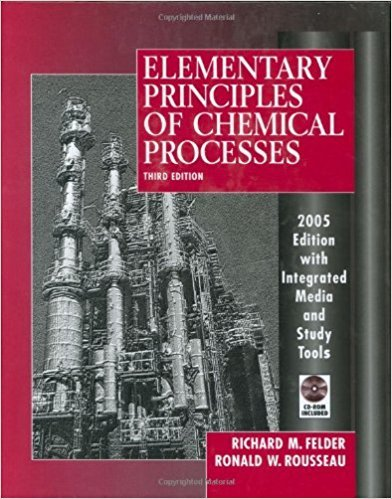×
Get Full Access to Elementary Principles Of Chemical Processes - 3 Edition - Chapter 2 - Problem 2.22
Get Full Access to Elementary Principles Of Chemical Processes - 3 Edition - Chapter 2 - Problem 2.22

×

# The Prandtl number, Npr, is a dimensionless groupISBN: 9780471687573 143

## Solution for problem 2.22 Chapter 2

Elementary Principles of Chemical Processes | 3rd Edition

• Textbook Solutions
• 2901 Step-by-step solutions solved by professors and subject experts
• Get 24/7 help from StudySoup virtual teaching assistantsElementary Principles of Chemical Processes | 3rd Edition

4 5 1 413 Reviews
16
1
Problem 2.22

The Prandtl number, Npr, is a dimensionless group important in heat transfer calculations. It is defined as Cpf-L/ k, where Cp is the heat capacity of a fluid, f-L is the fluid viscosity, and k is the thermal conductivity. For a particular fluid, Cp = 0.583 J/(g'0C), k = 0.286 W/(m.0C), and f-L = 1936 lbm/(fth). Estimate the value of NPr without using a calculator (remember, it is dimensionless), showing your calculations; then determine it with a calculator.

Step-by-Step Solution:
Step 1 of 3

Chapter 17: Aspects of Aqueous Equilibria Strong electrolytes exist as separate ions in solution The Common-Ion Effect  Consider a solution of acetic acid  If acetate ion, CH COO , is added to the solution, LeChatelier says the 3 equilibrium will shift to the left, meaning the formation of acetic acid will be favored and the H concentration will decrease  “The extent of ionization of a weak electrolyte is decreased by adding a strong electrolyte to solution that has an ion in common with the weak electrolyte”  Common ion equilibrium problems are solved similarly to other equilibrium problems (ICE tables)  However, the initial concentration of the common ion (from the salt) must be considered Buf

Step 2 of 3

Step 3 of 3

##### ISBN: 9780471687573

Unlock Textbook Solution